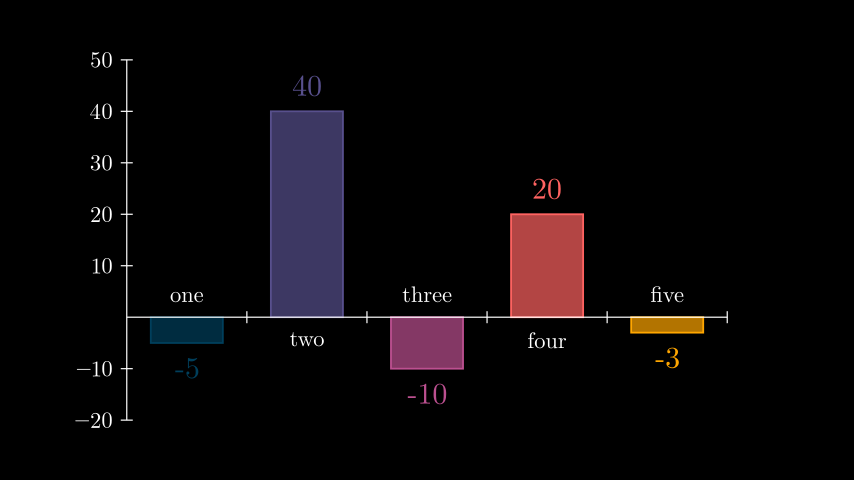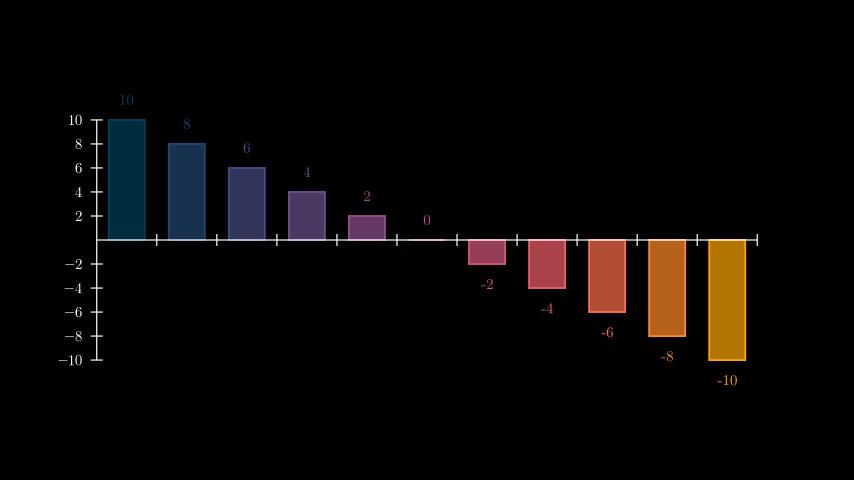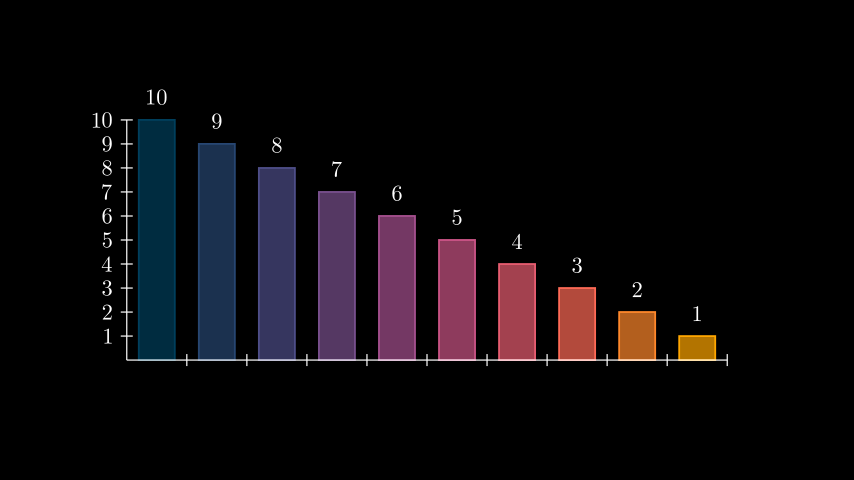# BarChart#

Qualified name: `manim.mobject.graphing.probability.BarChart`

class BarChart(values, bar_names=None, y_range=None, x_length=None, y_length=None, bar_colors=['#003f5c', '#58508d', '#bc5090', '#ff6361', '#ffa600'], bar_width=0.6, bar_fill_opacity=0.7, bar_stroke_width=3, **kwargs)[source]#

Bases: `Axes`

Creates a bar chart. Inherits from `Axes`, so it shares its methods and attributes. Each axis inherits from `NumberLine`, so pass in `x_axis_config`/`y_axis_config` to control their attributes.

Parameters
• values (MutableSequence[float]) – A sequence of values that determines the height of each bar. Accepts negative values.

• bar_names (Sequence[str] | None) – A sequence of names for each bar. Does not have to match the length of `values`.

• y_range (Sequence[float] | None) – The y_axis range of values. If `None`, the range will be calculated based on the min/max of `values` and the step will be calculated based on `y_length`.

• x_length (float | None) – The length of the x-axis. If `None`, it is automatically calculated based on the number of values and the width of the screen.

• y_length (float | None) – The length of the y-axis.

• bar_colors (Iterable[str]) – The color for the bars. Accepts a sequence of colors (can contain just one item). If the length of``bar_colors`` does not match that of `values`, intermediate colors will be automatically determined.

• bar_width (float) – The length of a bar. Must be between 0 and 1.

• bar_fill_opacity (float) – The fill opacity of the bars.

• bar_stroke_width (float) – The stroke width of the bars.

Examples

Example: BarChartExample```from manim import *

class BarChartExample(Scene):
def construct(self):
chart = BarChart(
values=[-5, 40, -10, 20, -3],
bar_names=["one", "two", "three", "four", "five"],
y_range=[-20, 50, 10],
y_length=6,
x_length=10,
x_axis_config={"font_size": 36},
)

c_bar_lbls = chart.get_bar_labels(font_size=48)

```

Methods

 `change_bar_values` Updates the height of the bars of the chart. `get_bar_labels` Annotates each bar with its corresponding value.

Attributes

 `animate` Used to animate the application of any method of `self`. `animation_overrides` `color` `depth` The depth of the mobject. `fill_color` If there are multiple colors (for gradient) this returns the first one `height` The height of the mobject. `n_points_per_curve` `sheen_factor` `stroke_color` `width` The width of the mobject.
change_bar_values(values, update_colors=True)[source]#

Updates the height of the bars of the chart.

Parameters
• values (Iterable[float]) – The values that will be used to update the height of the bars. Does not have to match the number of bars.

• update_colors (bool) – Whether to re-initalize the colors of the bars based on `self.bar_colors`.

Examples

Example: ChangeBarValuesExample```from manim import *

class ChangeBarValuesExample(Scene):
def construct(self):
values=[-10, -8, -6, -4, -2, 0, 2, 4, 6, 8, 10]

chart = BarChart(
values,
y_range=[-10, 10, 2],
y_axis_config={"font_size": 24},
)

chart.change_bar_values(list(reversed(values)))
```
get_bar_labels(color=None, font_size=24, buff=0.25, label_constructor=<class 'manim.mobject.text.tex_mobject.Tex'>)[source]#

Annotates each bar with its corresponding value. Use `self.bar_labels` to access the labels after creation.

Parameters
• color (Color | None) – The color of each label. By default `None` and is based on the parent’s bar color.

• font_size (float) – The font size of each label.

• buff (float) – The distance from each label to its bar. By default 0.4.

• label_constructor (type[VMobject]) – The Mobject class to construct the labels, by default `Tex`.

Examples

Example: GetBarLabelsExample```from manim import *

class GetBarLabelsExample(Scene):
def construct(self):
chart = BarChart(values=[10, 9, 8, 7, 6, 5, 4, 3, 2, 1], y_range=[0, 10, 1])

c_bar_lbls = chart.get_bar_labels(
color=WHITE, label_constructor=MathTex, font_size=36
)